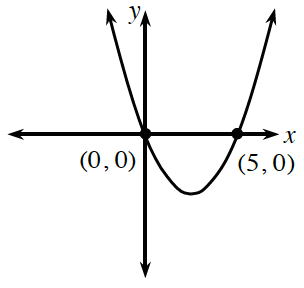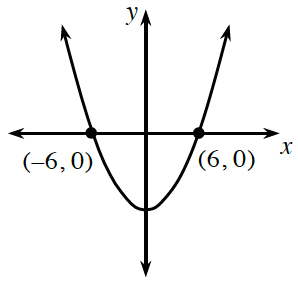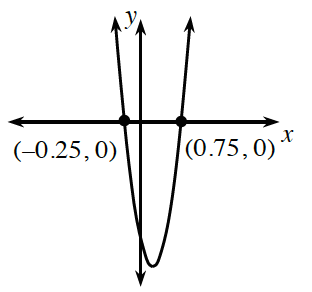### Home > INT2 > Chapter 6 > Lesson 6.2.2 > Problem6-65

6-65.

Based on the graph, give a possible equation for the parabola.

1.1.1.If the $x$-intercepts are $(p, 0)$ and $(r, 0),$ then the equation of the parabola in factored form is $y = a(x - p)(x - r)$, where $a$ is the stretch factor.

$y = a(x - 0)(x - 5)$